## Filters

Sort by :
Clear All
Q

3.20 b) Given the resistances of 1 Ω, 2 Ω, 3 Ω, how will be combine them to get an equivalent resistance of (iii) 6 Ω

1 Ω+2 Ω+ 3 Ω= 6 Ω, so we will combine the resistance in series.

Q 9.25  What should be the distance between the object in figure 9.23 and the magnifying glass if the virtual image of each square in the figure is to have an area of 6.25 mm2. Would you be able to see the squares distinctly with your eyes very close to the magnifier?

Given Virtual image area = 6.25 mm2 actual ara = 1 mm2 We can calculate linear magnification as  we also know  NOW, ACCORDING TO THE LENS FORMULA  and  since the image is forming at a distance which is less than 25 cm, it can not be seen by eye distinctively.

Q 9.24     (a)  At what distance should the lens be held from the figure  9.29(9.23 in latest edition) in order to view the squares distinctly with the maximum possible magnifying power?

(b) What is the magnification in this case?

(c) Is the magnification equal to the magnifying power in this case?

a) maximum magnifying is possible when our image distance will be equal to minimum vision point that is,      (Given) Now According to the lens formula Hence required object distance for viewing squares distinctly is 7.14 cm away from the lens. b) Magnification of the lens:   c) Magnifying power    since the image is forming at near point ( d = 25 cm ), both magnifying power and...

Q 9.32  Figure 9.34 shows an equiconvex lens (of refractive index 1.50) in contact with a liquid layer on top of a   plane mirror. A small needle with its tip on the principal axis is moved along the axis until its inverted image is found at the position of the needle. The distance of the needle from the lens is measured to be 45.0cm. The liquid is removed and the experiment is repeated. The new distance is measured to be 30.0cm. What is the refractive index of the liquid?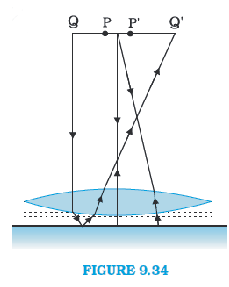Given The focal length of the convex lens  here liquid is acting like the mirror so, the focal length of the liquid  the focal length of the system(convex + liquid)  Equivalent focal length when two optical systems are in contact   NOW, let us assume refractive index of the lens be  The radius of curvature are  and . As we know, Now, let refractive index of liquid be  The radius of...

Q 9.31     Light incident normally on a plane mirror attached to a galvanometer coil retraces backwards as shown in Fig. 9.33. A current in the coil produces a deflection of 3.5o of the mirror. What is the displacement of the reflected spot of light on a screen placed 1.5 m away?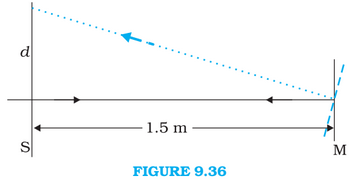Given Angle of deflection  the distance of the screen from the mirror  the reflected rays will bet deflected by twice angle of deviation that is   Now from the figure, it can be seen that Hence displacement of the reflected spot of the light is .

Q9.30  A Cassegrain telescope uses two mirrors as shown in Fig. 9.30. Such a telescope is built with the mirrors 20mm apart. If the radius of curvature of the large mirror is 220mm and the small mirror is 140mm, where will the final image of an object at infinity be?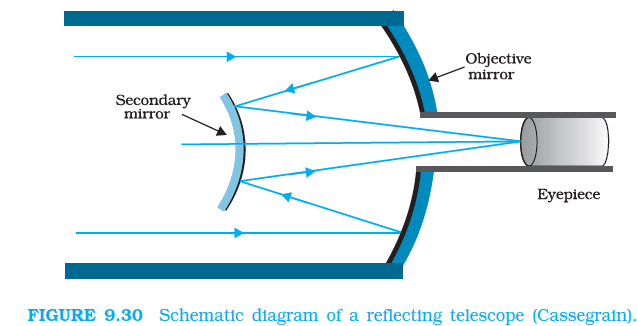Given, Distance between the objective mirror and secondary mirror  The radius of curvature of the Objective Mirror    so Focal length of the objective mirror   The radius of curvature of the secondary mirror    so, the Focal length of the secondary mirror   The image of an object which is placed at infinity, in the objective mirror, will behave like a virtual object for the secondary...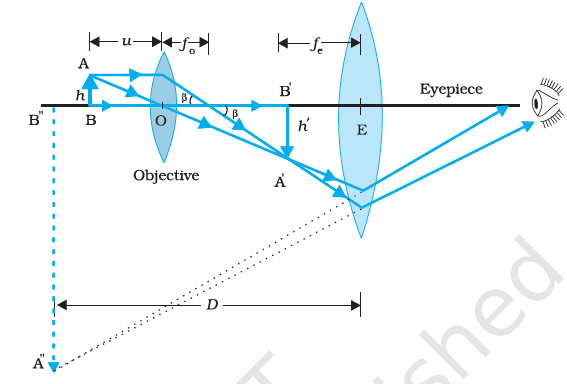Q 9.26    (c)     What is the height of the final image of the tower if it is formed at 25cm?

Given, image is formed at a distance  = 25cm as we know, magnification of eyepiece lens is given by : NOW,  height of the final image is given by : Therefore, the height of the final image will be 28.2 cmQ 9.26    (b) If this telescope is used to view a 100 m tall tower 3 km away, what is the height of the image of the tower formed by the objective lens?

Given, focal length of the objectove lens = = 140cm focal length of the eyepiece lens =  = 5 cm Height of tower  = 100m Distance of object which is acting like a object  = 3km = 3000m. The angle subtended by the tower at the telescope  NOW, let the height of the image of the tower by the objective lens is  . angle made by the image by the objective lens  : Since both, the angles are the same...

Q. 13.31     Suppose India had a target of producing by 2020 AD, 200,000 MW of electric power, ten percent of which  was to be obtained from nuclear power plants. Suppose we are given that, on an average, the efficiency of utilization (i.e. conversion to electric energy) of thermal energy produced in a reactor was 25%. How much amount of fissionable uranium would our country need per year by 2020? Take the heat energy per fission of 235U to be about 200MeV.

Let the amount of Energy to be produced using nuclear power per year in 2020 is E      (Only 10% of the required electrical energy is to be produced by Nuclear power and only 25% of                                                                                                         thermo-nuclear is successfully converted into electrical energy) Amount of Uranium required to produce this...

Q. 13.30     Calculate and compare the energy released by a) fusion of 1.0 kg of hydrogen deep within Sun and b) the fission of 1.0 kg of 235U in a fission reactor.

(a)   The above fusion reaction releases the energy of 26 MeV Number of Hydrogen atoms in 1.0 kg of Hydrogen is 1000NA Therefore 250NA such reactions would take place The energy released in the whole process is E1 (b) The energy released in fission of one  atom is 200 MeV Number of  atoms present in 1 kg of  is N The energy released on fission of N atoms is E2

Q. 13.29     Obtain the maximum kinetic energy of - particles, and the radiation frequencies of  decays in the decay                    scheme shown in Fig. 13.6. You are given that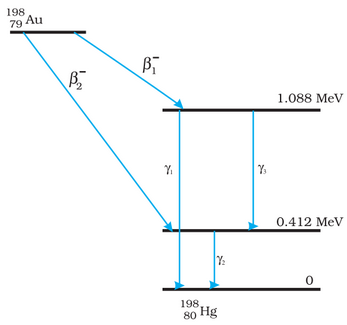decays from 1.088 MeV to 0 V Frequency of  is  Plank's constant, h=6.6210-34 Js  Similarly, we can calculate frequencies of  and  The energy of the highest level would be equal to the energy released after the decay Mass defect is We know 1u = 931.5 MeV/c2 Q value= 0.001473931.5=1.3721 MeV The maximum Kinetic energy of  would be 1.3721-1.088=0.2841 MeV The maximum Kinetic energy of  would be...

Q.13.28     Consider the D–T reaction (deuterium–tritium fusion)

(b) Consider the radius of both deuterium and tritium to be approximately 2.0 fm. What is the kinetic energy needed to overcome the coulomb repulsion between the two nuclei? To what temperature must the gas be heated to initiate the reaction? (Hint: Kinetic energy required for one fusion event =average thermal kinetic energy available with the interacting particles ; k = Boltzman’s constant, T = absolute temperature.)

To initiate the reaction both the nuclei would have to come in contact with each other. Just before the reaction the distance between their centres would be 4.0 fm. The electrostatic potential energy of the system at that point would be The same amount of Kinetic Energy K would be required to overcome the electrostatic forces of repulsion to initiate the reaction It is given that  Therefore...

Q.13.28     Consider the D–T reaction (deuterium-tritium fusion)

(a) Calculate the energy released in MeV in this reaction from the data:

The mass defect of the reaction is  1u = 931.5 MeV/c2 Q=0.018883931.5=17.59 MeV

Q.13.27     Consider the fission of by fast neutrons. In one fission event, no neutrons are emitted and the final end products, after the beta decay of the primary fragments, are  and . Calculate Q   for this fission process. The relevant atomic and particle masses are

The fission reaction given in the question can be written as The mass defect for the above reaction would be In the above equation, mN represents nuclear masses but 1u =931.5 MeV/c2 Q=0.247995931.5 Q=231.007 MeV Q value of the fission process is 231.007 MeVQ 9.29     (a) For the telescope described in Figure what is the separation between the objective lens and the eyepiece?

a) Given, focal length of the objective lens = = 140cm focal length of the eyepiece lens =  = 5 cm  The separation between the objective lens and eyepiece lens is given by: Hence, under normal adjustment separation between two lenses of the telescope is 145 cm.

Q 9.28  A small telescope has an objective lens of focal length 140cm and an eyepiece of focal length 5.0cm. What is the magnifying power of the telescope for viewing distant objects when

(b) the final image is formed at the least distance of distinct vision (25cm)?

Given, the focal length of the objective lens  the focal length of the eyepiece lens  normally, least distance of vision = 25cm NOW, as we know magnifying power when the image is at d = 25 cm is Hence magnification, in this case, is 33.6.

Q 9.28  A small telescope has an objective lens of focal length 140cm and an eyepiece of focal length 5.0cm. What  is the magnifying power of the telescope for viewing distant objects when
(a) the telescope is in normal adjustment (i.e., when the final image is at infinity)?

Given, the focal length of the objective lens  the focal length of the eyepiece lens  normally, least distance of vision = 25cm NOW, as we know magnifying power:   Hence magnifying power is 28.

Q9.27     An angular magnification (magnifying power) of 30X is desired using an objective of focal length 1.25cm and an eyepiece of focal length 5cm. How will you set up the compound microscope?

Given, magnifying power = 30 objective lens focal length = 1.25cm eyepiece lens focal length = 5 cm Normally, image is formed at distance d = 25cm NOW, BY FORMULA; Angular magnification by eyepiece:    from here, magnification by the objective lens :      since   ( ) According to the lens formula: from here, hence object must be 1.5 cm away from the objective lens. NOW FOR THE EYEPIECE...